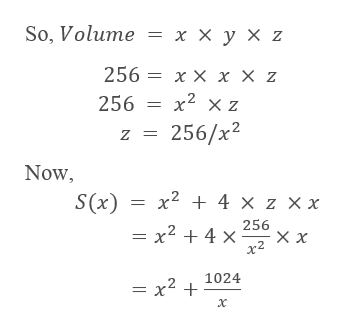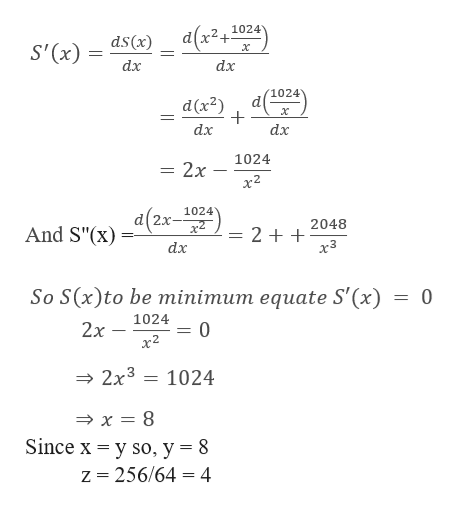# A crate open at the top has vertical sides, a square bottom, and a volume of 256 cubic meters. Using the labels shown in the figure, write the surface area S as a function of x. S(x) =  If the crate has the least possible surface area, find its dimensions.x = metersy = metersz = metersS"(x) = (value for your solution for x)

Question
11 views

A crate open at the top has vertical sides, a square bottom, and a volume of 256 cubic meters. Using the labels shown in the figure, write the surface area S as a function of x.

S(x) =

If the crate has the least possible surface area, find its dimensions.

x = meters
y = meters
z = meters
S"(x) = (value for your solution for x)

check_circle

Step 1

Given,

A crate open at the top has vertical sides, a square bottom, and a volume of 256 cubic meters.

Let the length of the side of the bottom square be x m then

Let breadth of the bottom square be y m.

So, x = y

Let height of the cube be z in metershelp_outlineImage TranscriptioncloseSo, Volume %3ххухz 256 x x x X z 256 3D х2 х z 256/x2 Z = Now S(x) 3D х? + 4 X Z XX — х2 + 4х 256 хх х2 1024 — х2 + х fullscreen
Step 2

Now,

...help_outlineImage Transcriptionclosea(x2074) ds(x) S' (x) dx dx d(x2) х dx dx 1024 = 2x х2 _a(2x-1024) 2048 And S"(x) 2 x3 dx So S(x)to be minimum equate S' (x) = 0 1024 2x 0 х2 2x3 1024 x 8 Since x y so, y = 8 z 256/64 4 fullscreen

### Want to see the full answer?

See Solution

#### Want to see this answer and more?

Solutions are written by subject experts who are available 24/7. Questions are typically answered within 1 hour.*

See Solution
*Response times may vary by subject and question.
Tagged in

### Derivative